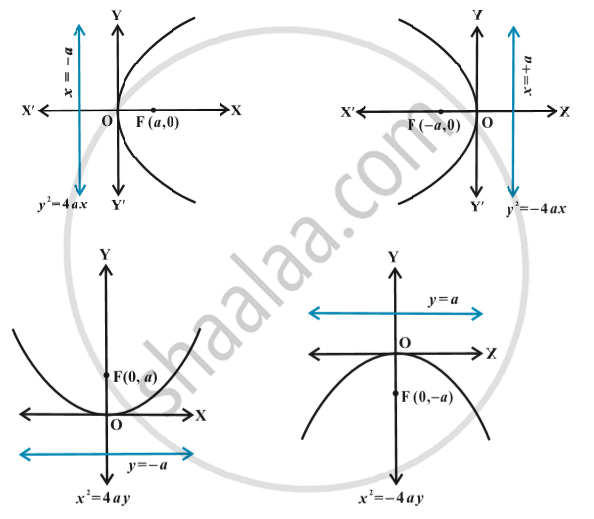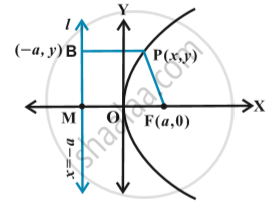# Parabola - Standard Equations of Parabola

## Notes

The equation of a parabola is simplest if the vertex is at the origin and the axis of symmetry is along the x-axis or y-axis. The four possible such orientations of parabola are shown in following fig.We will derive the equation for the parabola shown above in First  Fig with focus at (a, 0) a > 0; and directricx  x = – a as below:Let F be the focus and l the directrix.  Let FM be perpendicular to the directrix and bisect FM at the point O.  Produce MO to X.  By the definition of  parabola, the mid-point O is on the parabola and is called the vertex of the parabola. Take O as origin, OX the x-axis and OY perpendicular to it as the y-axis.  Let the distance from the directrix to the focus be 2a. Then, the coordinates of the focus are (a, 0),  and the equation of the directrix is x + a = 0 as in above fig.
Let P(x, y) be any point on the parabola such that
PF = PB,                                      ... (1)
where PB is perpendicular to l. The coordinates of B are (– a, y).
By the distance formula, we have
PF = sqrt((x-a)^2+y^2) and PB = sqrt(x + a)^2
Since PF = PB, we have
sqrt((x-a)^2 + y^2) = sqrt((x+a)^2)
i.e. (x – a)^2 + y^2 = (x + a)^2

or x^2 – 2ax + a^2 + y^2 = x^2 + 2ax + a^2
or y^2 = 4ax ( a > 0).
Hence, any point on the parabola satisfies
y^2 = 4ax.                    ...(2)
Conversely, let P(x, y) satisfy the equation (2)
PF = sqrt((x-a)^2+y^2) = sqrt((x-a)^2 + 4ax)
= sqrt(x+a)^2 = PB         .....(3)
and  so P(x,y) lies  on the parabola.
Standard equation of Parabola:
y^2 = 4ax
y^2 = - 4ax
x^2 = 4ay
x^2 = - 4ay

If you would like to contribute notes or other learning material, please submit them using the button below.

### Shaalaa.com

Standard equation of parabola [00:08:12]
S
0%